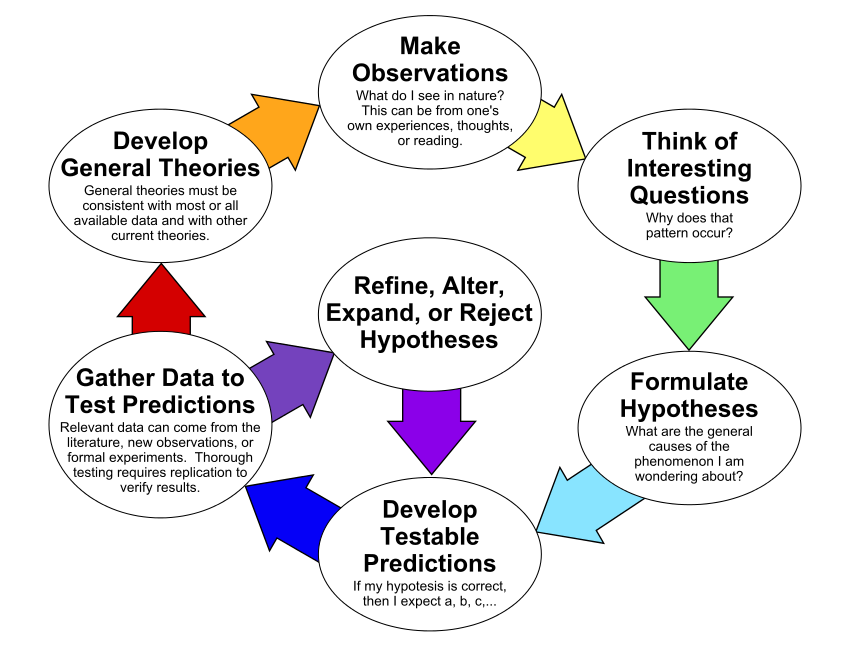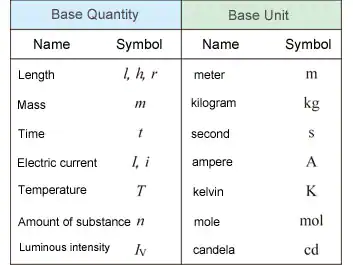# Intro to Chemistry!

What is chemistry?

Chemistry is the branch of science that studies matter, what it is and how it changes.

Matter - anything that occupies space and has mass

Everything we see, touch, smell, and taste involves chemistry and chemicals, which are all matter. Chemistry investigates the properties of matter, how they interact and how they change.

Chemistry is everywhere...

examples:

cooking - the creation of food; how and why food rots

cleaning - the creation and use of detergents, disinfectants, and soaps

medicine - the creation and use of drugs, vitamins, and supplements

environment - the creation and spreading of pollutants and the creation of materials to clean up and prevent pollution

Scientific Method:

1. Ask a question or identify a problem

2. Conduct background research

3. Create a hypothesis (if false change variable and begin again)

4. Test the hypothesis with experiments

5. Make observations and collect data

6. Analyze results and draw conclusions (if false change variable and begin again)

7. Share resultsThis process isn't always straightforward. Scientists often find themselves coming back to the same questions again and again.

Scientists use pure science and applied science to conduct scientific investigations.

Pure Science - the search for knowledge or facts; it uses theories and predictions to understand nature; ex. geology

Applied Science - using knowledge in a practical way; related to engineering and technology; ex. the development of a rocket

Model - a representation of a particular situation using something else to represent it; it allows the scientist to easily observe and gather data

Physical Model - something that can be built such as a molecule that is made of marshmallows, gumdrops, and sticks

Computer Model - a three-dimensional simulation of a moving object or a chemical reaction

Mathematical Model - calculations involving a particular mathematical equation; ex. and equation of a line

Theory - a proposed explanation that is based on an examination of facts; facts can be observed and measure

Theories can be proven or rejected. They can also be changed and improved as more facts are gathered through experimentation or modeling. They are the basis for scientific knowledge and are a way to take collected facts and put them to practical use.

Law - a rule-based on observation of a process in nature that behaves the same way each and every time

A law describes what happens while a theory describes why something happens.

How to design a scientific experiment...

1. Observe something about which you are curious

2. Construct a hypothesis

3. Plan out the experiment to test the hypothesis

4. Predict the outcome

5. Conduct the experiment

6. Record the results

7. Repeat past experiments to see if you get the same results

An experiment requires a procedure and a list of materials and methods needed to conduct the experiment.

Procedure - a step by step list of how to carry out the experiment

You can have a controlled experiment by running the experiment more than once, first without changing any factors (this experiment is called the control) and then a second time, changing only the factor you want to observe. In a controlled experiment, the factors that are not changed are called constants and they don't affect the outcome of the experiment. A variable is a factor that can alter your experiment's results. A controlled experiment allows you to test the influence of the variable.

Independent - the variable that you change in an experiment

Dependent - the variable that is influenced by the independent variable; the results of your experiments

The International System of Units or SI System is the preferred method of measurement in chemistry. SI is an abbreviation for the french term systeme internationale which translates to international system.Dimensional Analysis - a mathematical method used to convert actual measured units into the units needed for the answer to a problem

Conversion Factor - is the relationship between the two units also known as a ratio

Measurements in IS...

Length - meter: distance between two points

Volume - liter: the amount of space that something occupies

Mass - gram: the amount of matter in a solid, liquid, or gas

Weight - newton: the force exerted by a mass by a gravitational field

Mass and weight are not the same

Mass is the amount of matter in an object and weight relies on gravity.

Weight = (mass) x (gravity) or W=mg

Time - seconds: period between events

Density - grams: amount of mass per unit volume

Temperature - kelvin: the measure of the average kinetic energy of the atoms or molecules in a system

Heat - calorie: the total energy of the molecular motion in a substance

Pressure - a measure of the force exerted on a unit area of surface

Standard atmospheric pressure - 1 atm, 1.013 x 10^5 Pa pr 101.3k Pa

The most important rule to remember in a chemistry lab is safety first.